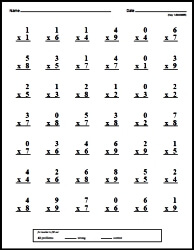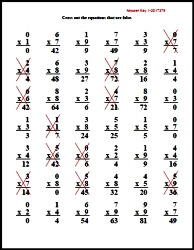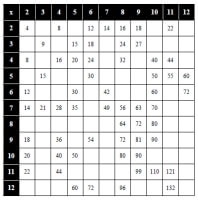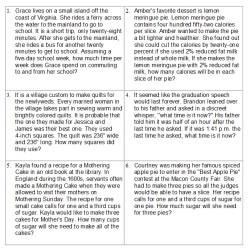edHelper.com Math Worksheets Multiplication Worksheets 6th Grade MultiplicationWorksheets, Lessons, and Printables

Math Facts

Quick Math FactsMake Quick Math Facts PrintableMultiplication Table and ChartMultiplication Table and Chart

Multiplication Word ProblemsMultiplication Word Problems (3rd grade) Multiplication Word Problems (4th grade) Multiplication Word Problems (5th grade) Multiplication Word Problems (6th grade)

Multiplication
Column Multiplication with Extra Space To Solve Problems
1-2 digits by 1 digit column multiplication
2 digits (and increasing 1 digit every 2 rows) by 1 digit column multiplication
2 digits (and increasing 1 digit every row) by 1 digit column multiplication
Multiples of 10 multiplication (2-5 digits by 1 digit)
2 digits by 2 digits column multiplication
2-3 digits by 2 digits column multiplication
4-5 digits by 2 digits column multiplication
2 digits (and increasing 1 digit every 2 rows) by 2 digit column multiplication
2 digits (and increasing 1 digit every row) by 2 digit column multiplication

Column Multiplication (more problems with less space)
1-2 digits by 1 digit column multiplication
2 digits (and increasing 1 digit every 2 rows) by 1 digit column multiplication
2 digits (and increasing 1 digit every row) by 1 digit column multiplication
Multiples of 10 multiplication (2-5 digits by 1 digit)
2 digits by 2 digits column multiplication
2-3 digits by 2 digits column multiplication
4-5 digits by 2 digits column multiplication
2 digits (and increasing 1 digit every 2 rows) by 2 digit column multiplication
2 digits (and increasing 1 digit every row) by 2 digit column multiplication

Part 2
1-2 digits by 1 digit across multiplication
3-4 digits by 1 digit across multiplication
1-2 digits by 2 digits across multiplication
Across multiplication with 3-5 numbers
Fill in the missing numbers: 1-2 digits
Fill in the missing numbers: 2 digits
Fill in the missing numbers: 2-3 digits
Math multiplication boxes: 3 x 3 (numbers 1-9)
Math multiplication boxes: 4 x 4 (numbers 1-9)
Math multiplication boxes: 3 x 3 (numbers 4-19)
Multiplication word problems (basic)
Multiplication word problems
Multiplication word problems
Multiplication Mixed Review

Multiplying by 3-5 Digits
2-3 digits by 2-3 digits column multiplication
(extra space for work) 2-3 digits by 2-3 digits column multiplication
Multiples of 10 multiplication (2-5 digits by 1 digit)
(extra space for work) Multiples of 10 multiplication (2-5 digits by 1 digit)
3-4 digits by 3-4 digits column multiplication
(extra space for work) 3-4 digits by 3-4 digits column multiplication
2-5 digits by 2-5 digits column multiplication
(extra space for work) 2-5 digits by 2-5 digits column multiplication
Fill in the missing numbers: 3-4 digits
2-3 digits by 3-5 digits across multiplication
Across multiplication with 3-5 numbers (2 Digits)
Multiplication number word puzzles
Multiplication word problems

Review
Multiplication Mixed Review

Division Facts Through 10
Multiplication and Division: Fill in the missing numbers
Multiplication and division with 2
Multiplication and division with 3
Multiplication and division with 4
Multiplication and division with 5
Multiplication and division with 6
Multiplication and division with 7
Multiplication and division with 8
Multiplication and division with 9
Multiplication and division with 10
Multiplication and division (mix)

Multiply Decimals
Column Multiplication with Extra Space To Solve Problems
Multiply a decimal (up to hundredths) by a Whole Number
Multiply a decimal (up to ten-thousandths) by a Whole Number
Multiply 2 decimals (up to tenths; numbers are less than 1)
Multiply 2 decimals (up to hundredths; numbers are less than 1)
Multiply 2 decimals (up to thousandths; numbers are less than 1)
Multiply 2 decimals (numbers are from 0 to 999.99)

Column Multiplication (more problems with less space)
Multiply a decimal (up to hundredths) by a Whole Number
Multiply a decimal (up to ten-thousandths) by a Whole Number
Multiply 2 decimals (up to tenths; numbers are less than 1)
Multiply 2 decimals (up to hundredths; numbers are less than 1)
Multiply 2 decimals (up to thousandths; numbers are less than 1)
Multiply 2 decimals (numbers are from 0 to 999.99)

Part 2
Across multiplication: a decimal (up to hundredths) by a Whole Number
Fill in the missing numbers (up to tenths)
Fill in the missing numbers (up to hundredths)
Fill in the missing numbers (up to thousandths)
Order of Operations (no parenthesis; numbers up to 9.9)
Order of Operations (no parenthesis; numbers up to 99.99)
Order of Operations
Order of Operations (one missing number)
Order of Operations (one missing operation)
Order of Operations (fill in all numbers)
Order of Operations (fill in all operations)
Decimal multiplication word problems
Multiplication of decimals: Mixed Review

Solving Multiplication Equations
Solving multiplication and division equations
Solving addition, subtraction, multiplication, and division equations
Write an equation for each problem. Then solve the equation.

Multiply and Divide Fractions
Multiply fractions
Multiply fractions: find the value of y
Multiply fractions with mixed numbers
Multiply fractions with mixed numbers: find the value of y
Divide fractions
Divide fractions: find the value of y
Divide fractions with mixed numbers
Divide fractions with mixed numbers: find the value of y
Multiply and divide fractions
Multiply and divide fractions: find the value of y
Multiply and divide fractions with mixed numbers
Multiply and divide fractions with mixed numbers: find the value of y
Multiply and Divide Fractions Mixed Review

Beginning Algebra with Multiplication
Solving multiplication and division equations
Solving equations (mix)

More Decimals and Multiplication
Multiply decimals
Open-ended decimal questions
Decimals Word Problems Printable
Decimals Word Problems Printable: Science Problems (Physics, Biology, and Chemistry)
Decimals Word Problems Printable: Scientific Notation
Decimals Review Printable

Multiplication

Have a suggestion or would like to leave feedback?